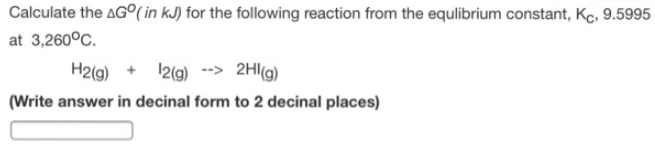# Problem: Calculate the ΔG° (in kJ) for the following reaction from the equlibrium constant, KC, 9.5995 at 3, 260°C. H2 (g) + I2 (g) → 2HI (g) (Write answer in decimal form to 2 decimal places)

###### FREE Expert Solution
83% (479 ratings)###### Problem Details

Calculate the ΔG° (in kJ) for the following reaction from the equlibrium constant, KC, 9.5995 at 3, 260°C.

H2 (g) + I2 (g) → 2HI (g)

(Write answer in decimal form to 2 decimal places)